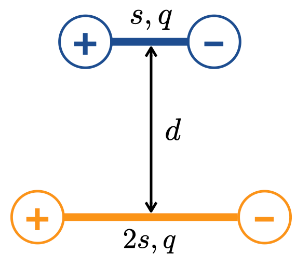# Question Solved1 AnswerTwo dipoles (blue and orange) are aligned down their middles asshown in the diagram. The dipoles’ charges and separations are alsoshown in the diagram. The distance between the centers of the twodipoles is d, which is much bigger than the dipoleseparations. Calculate the net electric field at a location exactlyhalfway between the centers of the two dipoles. 3,9 + - d + 2s, aTwo dipoles (blue and orange) are aligned down their middles as shown in the diagram. The dipoles’ charges and separations are also shown in the diagram. The distance between the centers of the two dipoles is d, which is much bigger than the dipole separations. Calculate the net electric field at a location exactly halfway between the centers of the two dipoles.Transcribed Image Text: 3,9 + - d + 2s, a
More
Transcribed Image Text: 3,9 + - d + 2s, a